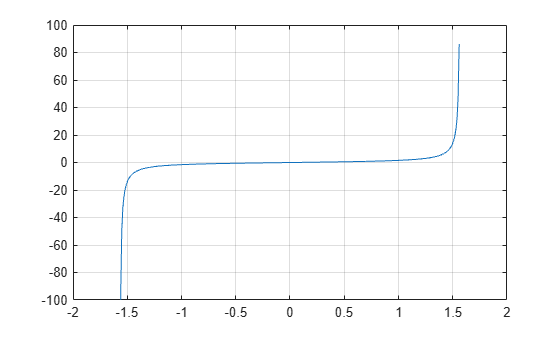Documentation

# tan

Tangent of argument in radians

## Syntax

``Y = tan(X)``

## Description

example

````Y = tan(X)` returns the tangent of each element of `X`. The `tan` function operates element-wise on arrays. The function accepts both real and complex inputs. For real values of `X`, `tan(X)` returns real values in the interval [-∞, ∞].For complex values of `X`, `tan(X)` returns complex values. ```

## Examples

collapse all

Plot the tangent function over the domain $-\pi /2\le x\le \pi /2$ .

```x = (-pi/2)+0.01:0.01:(pi/2)-0.01; plot(x,tan(x)), grid on```Calculate the tangent of the complex angles in vector `x`.

```x = [-i pi+i*pi/2 -1+i*4]; y = tan(x)```
```y = 1×3 complex 0.0000 - 0.7616i -0.0000 + 0.9172i -0.0006 + 1.0003i ```

## Input Arguments

collapse all

Input angle in radians, specified as a scalar, vector, matrix, or multidimensional array.

Data Types: `single` | `double`
Complex Number Support: Yes

## Output Arguments

collapse all

Tangent of input angle, returned as a real-valued or complex-valued scalar, vector, matrix or multidimensional array.

collapse all

### Tangent Function

The tangent of an angle, α, defined with reference to a right angled triangle is

.The tangent of a complex argument, α, is

`$\text{tan}\left(\alpha \right)=\frac{{e}^{i\alpha }-{e}^{-i\alpha }}{i\left({e}^{i\alpha }+{e}^{-i\alpha }\right)}\text{\hspace{0.17em}}.$`

.

## Tips

• In floating-point arithmetic, `tan` is a bounded function. That is, `tan` does not return values of `Inf` or `-Inf` at points of divergence that are multiples of `pi`, but a large magnitude number instead. This stems from the inaccuracy of the floating-point representation of π.